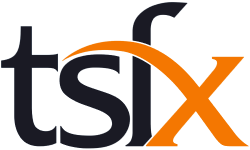# General Mathematics Course Catalogue

General Mathematics Units 3 and 4 focus on real-life application of mathematics and consist of the areas of study ‘Data analysis, probability and statistics’ and ‘Discrete mathematics’.

Unit 3 comprises Data analysis and Recursion and financial modelling, and Unit 4 comprises Matrices and Networks and decision mathematics.

Assumed knowledge and skills for General Mathematics Units 3 and 4 are contained in General Mathematics Units 1 and 2, and will be drawn on, as applicable, in the development of related content from the areas of study, and key knowledge and key skills for the outcomes of General Mathematics Units 3 and 4.

In undertaking these units, students are expected to be able to apply techniques, routines and processes involving rational and real arithmetic, sets, lists, tables and matrices, diagrams, networks, algorithms, algebraic manipulation, recurrence relations, equations and graphs. They should have facility with relevant mental and by-hand approaches to estimation and computation. The use of numerical, graphical, geometric, symbolic statistical and financial functionality of technology for teaching and learning mathematics, for working mathematically, and in related assessment, is to be incorporated throughout each unit as applicable.

## Course List

To filter the course list, enter your search text into the below field

For example, entering Unit 3 into the search field would show all Unit 3 General Mathematics Courses.
\Learnworlds\Codeneurons\Pages\ZoneRenderers\CourseCards